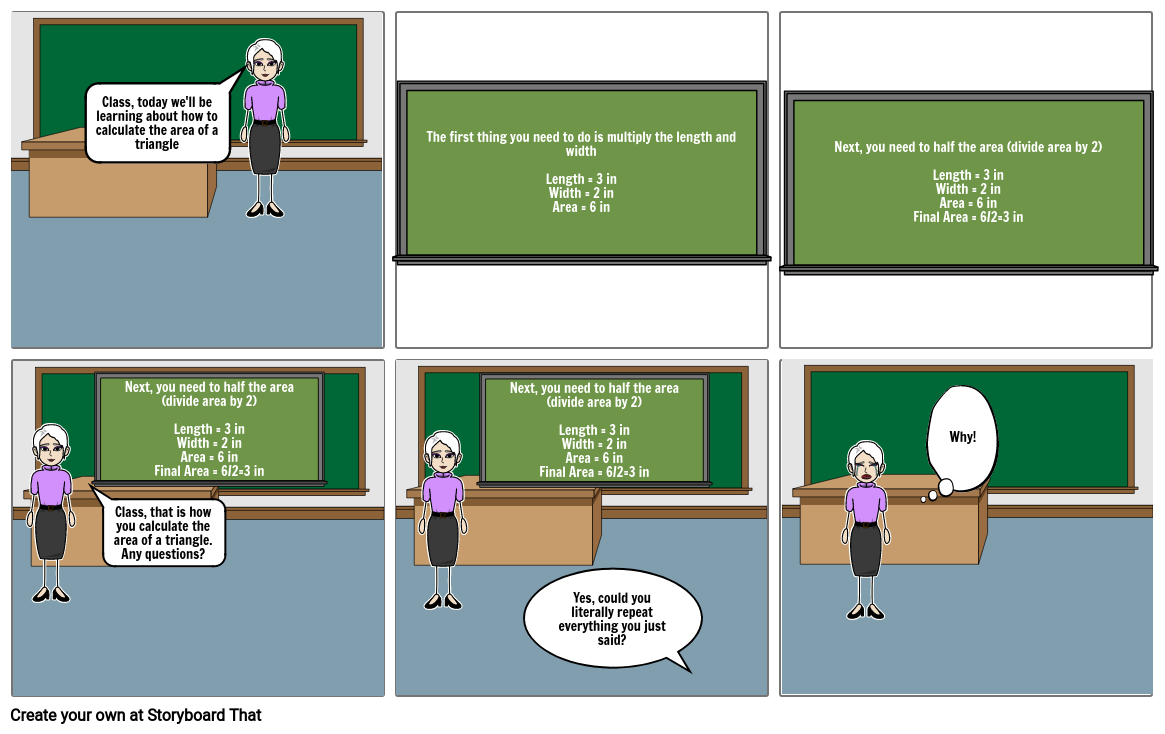Math
Updated: 5/22/2020This storyboard was created with StoryboardThat.com

#### Storyboard Text

• Class, today we'll be learning about how to calculate the area of a triangle
• The first thing you need to do is multiply the length and widthLength = 3 inWidth = 2 inArea = 6 in
• Next, you need to half the area (divide area by 2)Length = 3 inWidth = 2 inArea = 6 inFinal Area = 6/2=3 in
• Class, that is how you calculate the area of a triangle. Any questions?
• Next, you need to half the area (divide area by 2)Length = 3 inWidth = 2 inArea = 6 inFinal Area = 6/2=3 in
• Next, you need to half the area (divide area by 2)Length = 3 inWidth = 2 inArea = 6 inFinal Area = 6/2=3 in
• Yes, could you literally repeat everything you just said?
• Why!
##### Over 13 Million Storyboards Created